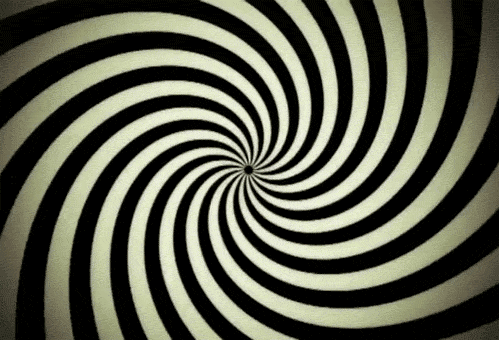# Square spiral make you giddy?Consider the rectangular spiral in the image below. It starts from the origin and twirls and twirls forever in an anticlockwise direction along the integer coordinates of the Cartesian coordinate plane.Each point along the spiral is numbered with an integer $Z$ as shown in the image below.What is the value of the integer $Z$ at the coordinate $(12,-22)$?

Details and assumptions

As an explicit example $Z$ is $10$ for $(2,0)$ , $3$ for $(0,1)$ and $10$ for $(2,0)$.

×

Problem Loading...

Note Loading...

Set Loading...